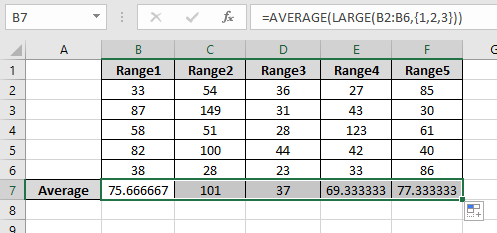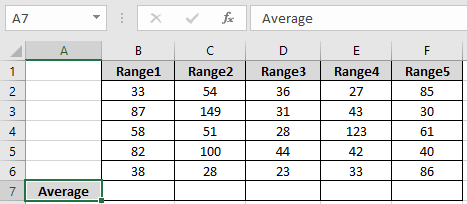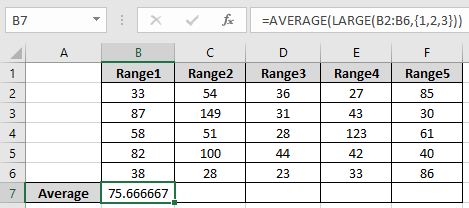# How to Find Average of Largest 3 Values from Last 5 Values in Microsoft Excel 2010In this article, we will learn How to Find Average of Largest 3 Values from Last 5 Values in Microsoft Excel 2010.

Scenario:

When working with data, where we need to get the average of numbers having criteria values. Why criteria values create a difference in result. Here given a situation were we need to extract the average of last 3 months data or last 3 years data. There we can use this formula. Understand how to solve the problem.

Formula Syntax:

To get the top 3 values in a list we will use the LARGE function.

The LARGE function returns the kth largest value starting from the largest in a range of values.

Syntax:

 =LARGE ( array, k )

Note: It will return #NUM! Error if k is greater than the number of values in the range.

AVERAGE function takes the array and returns the average value or mean value for the array.

Syntax:

 = AVERAGE ( number1 , number2 ,... )

Let's make a formula using the above functions:

Firstly, we will filter the array using the LARGE function and then the outcome will be fed to the AVERAGE function.

Syntax:

 = AVERAGE ( LARGE ( array , { 1 , 2, 3 } ) )

The above formula gets the first largest ,second largest and the third largest value from the array and fed to the AVERAGE function to get the result. Changing the values inside the burly braces will change the result.

Example :

All of these might be confusing to understand. Let's understand how to use the function using an example. This all might be confusing. So Let’s use the formula in an example to get its more understanding.

Here we have 5 ranges of numbers and We need to find the find the average of the top 3 values for all ranges.We need to find the average using the below formula

Use the formula:

 = AVERAGE ( LARGE ( B2:B6 , {1 , 2 , 3 } ) )

Explanation:

The LARGE function gets the top 3 values of the range ( B1:B6 ). The Large function returns the values { 87 , 82 , 58 }. Now AVERAGE of these values is calculated using the AVERAGE functionAs you can see the formula returns the average for first array

Now use the same formula for other ranges using the Ctrl + R or drag right option in excel.Here we have the average for all the ranges.

Here are all the observational notes regarding using the formula.

Notes:

1. The function returns #VALUE! criteria if no value matches the given criteria.
2. Different Criteria inside formula is accepted, such as equals to (=), less than (<) , less than or equals to (<=) , greater than or equals to (>=) , greater than (>) & not equals to (<>).

In this way, we learnt How to Find the Average of Largest 3 Values from Last 5 Values in Microsoft Excel 2010. You can use these functions in Excel 2016, 2013 and 2010. Find more articles on Mathematical operation and formulation with different criteria. If you liked our blogs, share it with your friends on Facebook. And also you can follow us on Twitter and Facebook. We would love to hear from you, do let us know how we can improve, complement or innovate our work and make it better for you. Write us at info@exceltip.com

Related Articles

How To Highlight Cells Above and Below Average Value : highlight values which are above or below the average value using the conditional formatting in Excel.

Ignore zero in the Average of numbers : calculate the average of numbers in the array ignoring zeros using AVERAGEIF function in Excel.

Calculate Weighted Average : find the average of values having different weight using SUMPRODUCT function in Excel.

Average Difference between lists : calculate the difference in average of two different lists. Learn more about how to calculate average using basic mathematical average formula.

Average numbers if not blank in Excel : extract average of values if cell is not blank in excel.

AVERAGE of top 3 scores in a list in excel : Find the average of numbers with criteria as highest 3 numbers from the list in Excel

Popular Articles:

How to use the IF Function in Excel : The IF statement in Excel checks the condition and returns a specific value if the condition is TRUE or returns another specific value if FALSE.

How to use the VLOOKUP Function in Excel : This is one of the most used and popular functions of excel that is used to lookup value from different ranges and sheets.

How to use the COUNTIF Function in Excel : Count values with conditions using this amazing function. You don't need to filter your data to count specific values. Countif function is essential to prepare your dashboard.

How to use the SUMIF Function in Excel : This is another dashboard essential function. This helps you sum up values on specific conditions.

1.Hi Hieu,

9.99999999999999E+307 is a biggest number in Excel.
In this example =MATCH(9.99999999999999E+307,A2:A10). Excel will lookup the last value in range.

Regards,
Ashish Mehra

•Hi Ashish Mehra,
I want to say thanks for you.

Regards,
Thanh Hieu

2.I don't understand why:
1-- passing for MATCH function 9.999999E+307
2-- the first parameter of LARGE function must array or range. So why we can use: "INDEX...:A10", because result of INDEX() is 6.